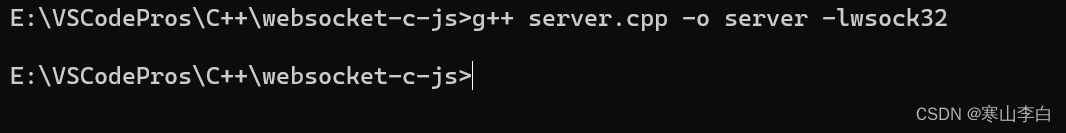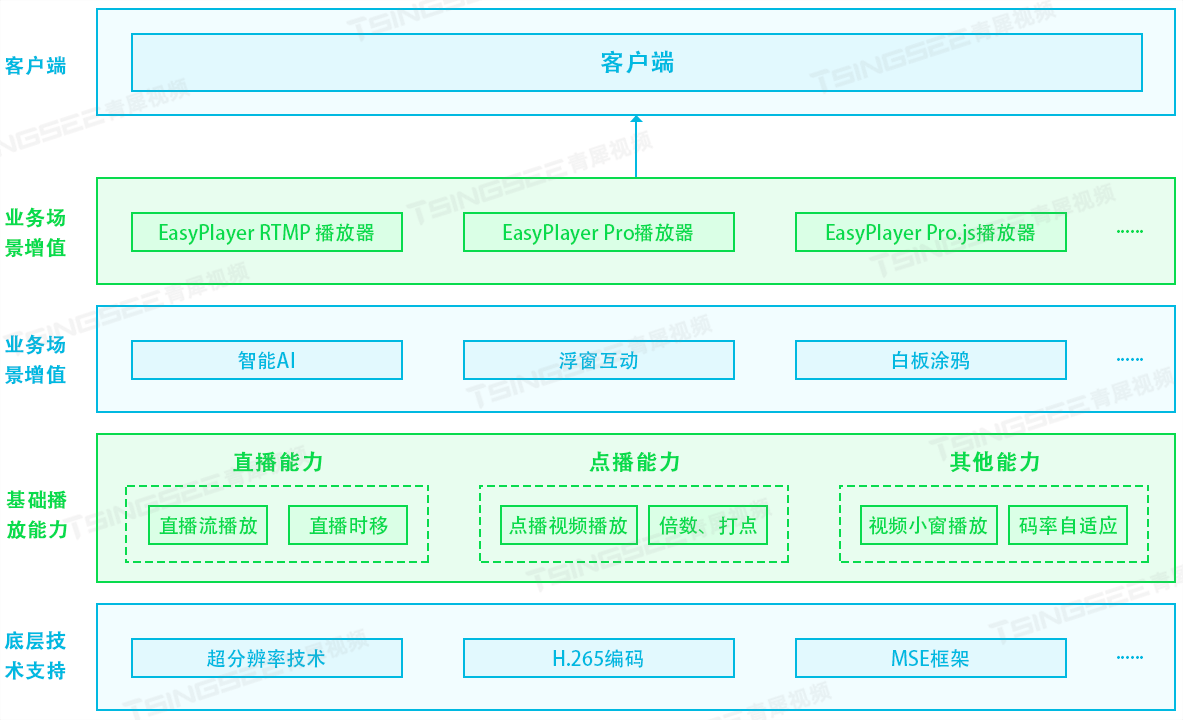# 数据清洗：数据挖掘的前期准备工作

⭐️⭐️⭐️⭐️⭐️欢迎来到我的博客⭐️⭐️⭐️⭐️⭐️
?作者：秋无之地

?简介：CSDN爬虫、后端、大数据领域创作者。目前从事python爬虫、后端和大数据等相关工作，主要擅长领域有：爬虫、后端、大数据开发、数据分析等。

?欢迎小伙伴们点赞??、收藏⭐️、留言?、关注?，关注必回关

## 一、数据清洗重要性

1、什么是数据清洗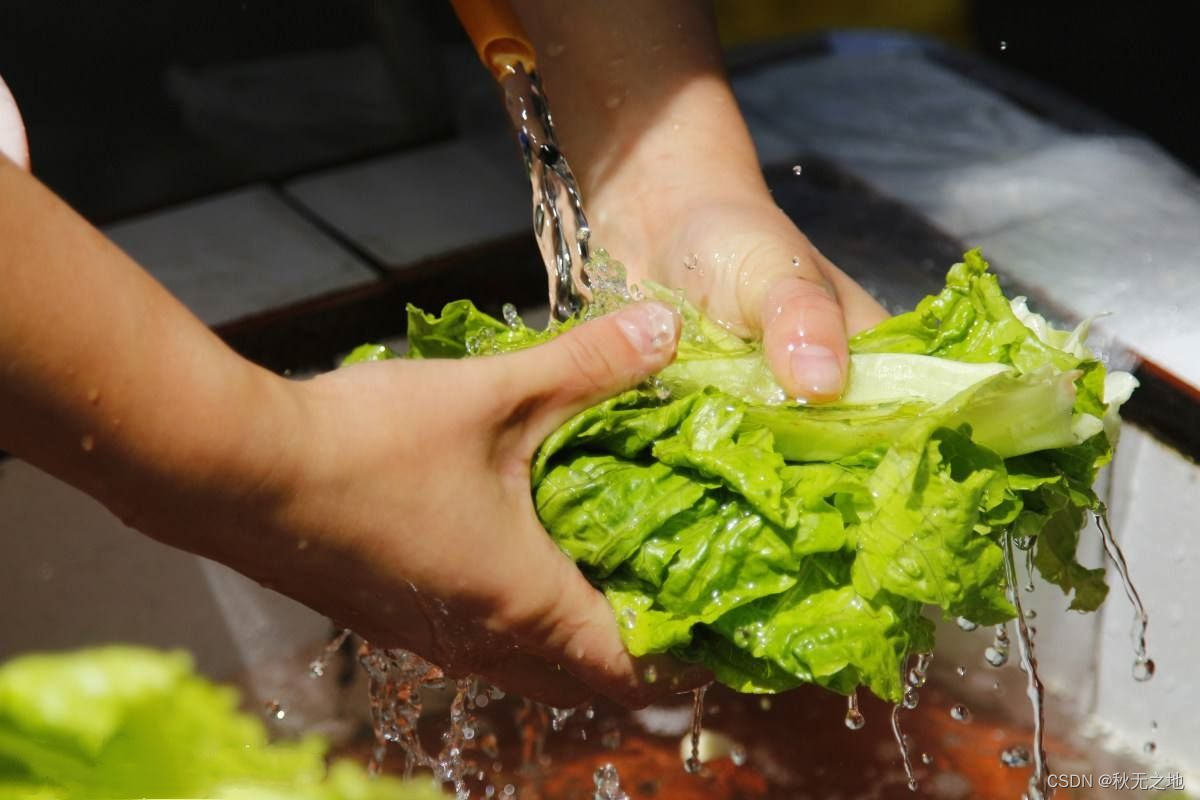文章来源地址:https://www.uudwc.com/A/R6ek8/

2、为什么要数据清洗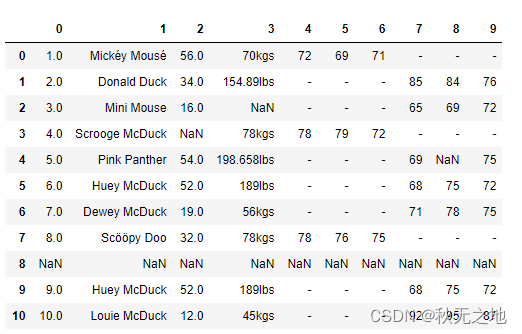## 二、数据质量的准则

1. 完整性：单条数据是否存在空值，统计的字段是否完善。
2. 全面性：观察某一列的全部数值，比如在 Excel 表中，我们选中一列，可以看到该列的平均值、最大值、最小值。我们可以通过常识来判断该列是否有问题，比如：数据定义、单位标识、数值本身。
3. 合法性：数据的类型、内容、大小的合法性。比如数据中存在非 ASCII 字符，性别存在了未知，年龄超过了 150 岁等。
4. 唯一性：数据是否存在重复记录，因为数据通常来自不同渠道的汇总，重复的情况是常见的。行数据、列数据都需要是唯一的，比如一个人不能重复记录多次，且一个人的体重也不能在列指标中重复记录多次。

1、完整性

• 删除：删除数据缺失的记录；
• 均值：使用当前列的均值；
• 高频：使用当前列出现频率最高的数据。

df['Age'].fillna(df['Age'].mean(), inplace=True)

age_maxf = train_features['Age'].value_counts().index
train_features['Age'].fillna(age_maxf, inplace=True)

# 删除全空的行
df.dropna(how='all',inplace=True) 

2、全面性

# 获取 weight 数据列中单位为 lbs 的数据
rows_with_lbs = df['weight'].str.contains('lbs').fillna(False)
# 将 lbs转换为 kgs, 2.2lbs=1kgs
for i,lbs_row in df[rows_with_lbs].iterrows():
# 截取从头开始到倒数第三个字符之前，即去掉lbs。
weight = int(float(lbs_row['weight'][:-3])/2.2)
df.at[i,'weight'] = '{}kgs'.format(weight) 

3、合理性

# 删除非 ASCII 字符
df['first_name'].replace({r'[^\x00-\x7F]+':''}, regex=True, inplace=True)
df['last_name'].replace({r'[^\x00-\x7F]+':''}, regex=True, inplace=True)

4、唯一性

# 切分名字，删除源数据列
df[['first_name','last_name']] = df['name'].str.split(expand=True)
df.drop('name', axis=1, inplace=True)

# 删除重复数据行
df.drop_duplicates(['first_name','last_name'],inplace=True)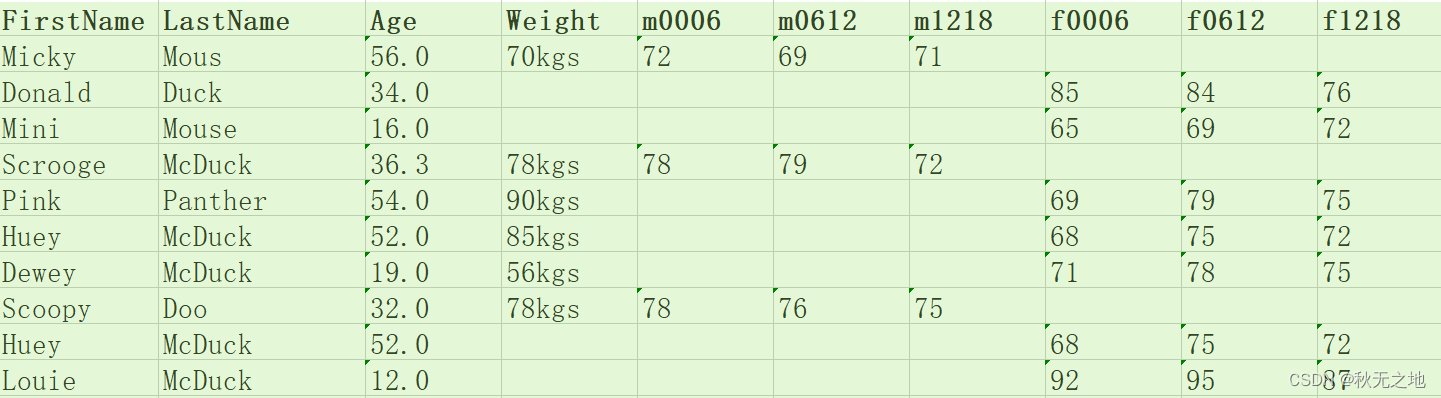## 四、总结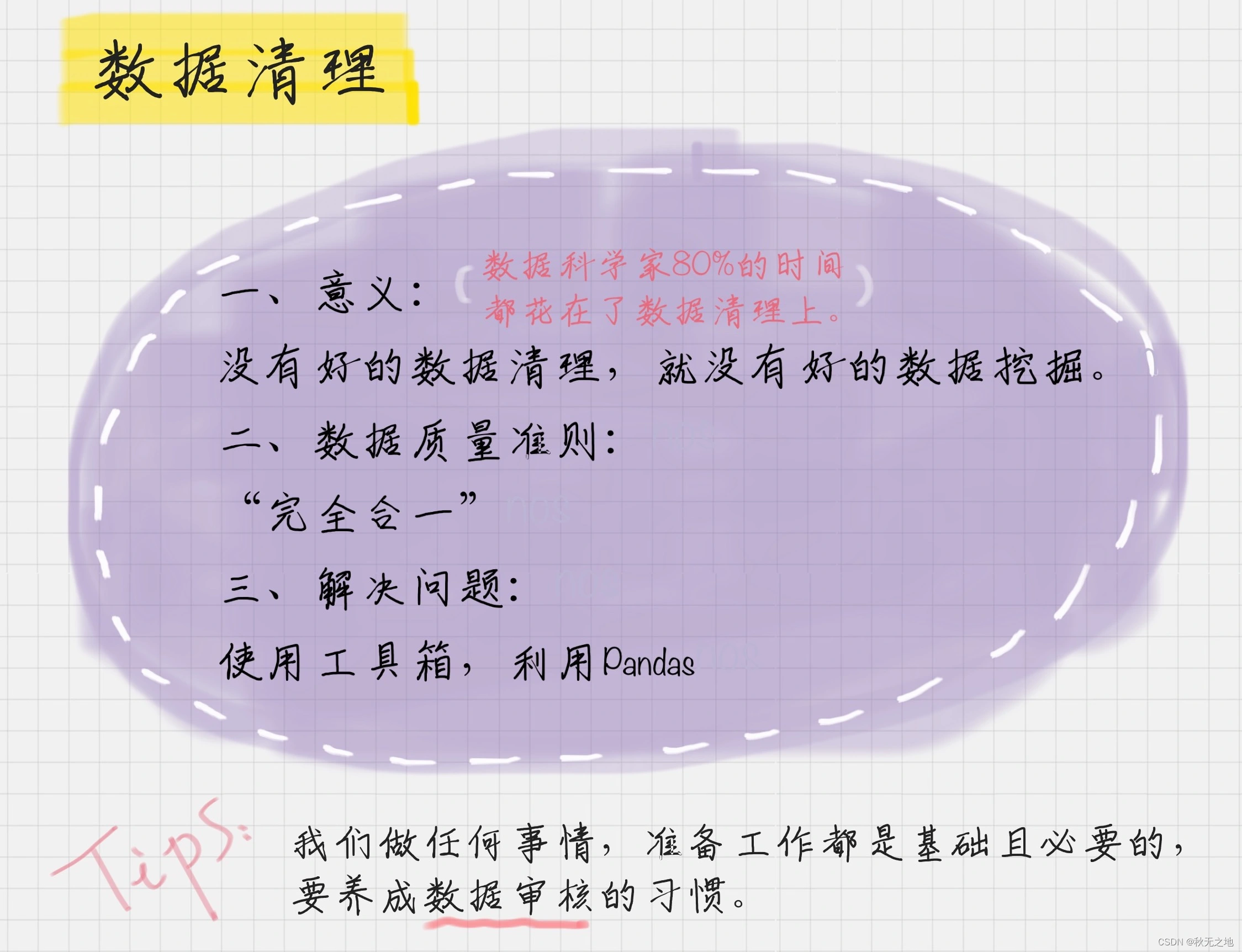## 版权声明